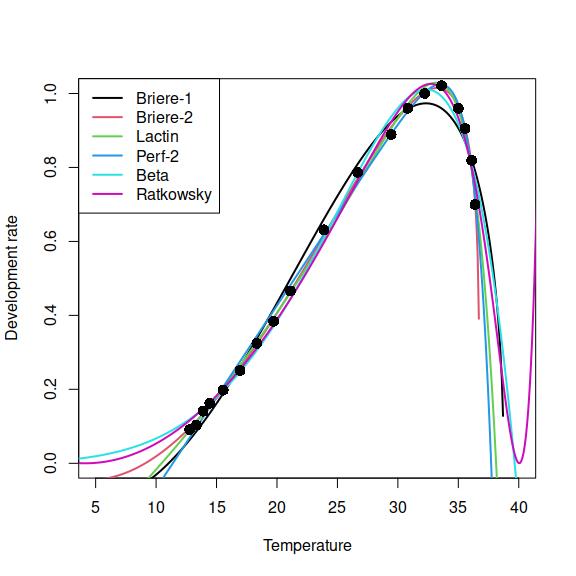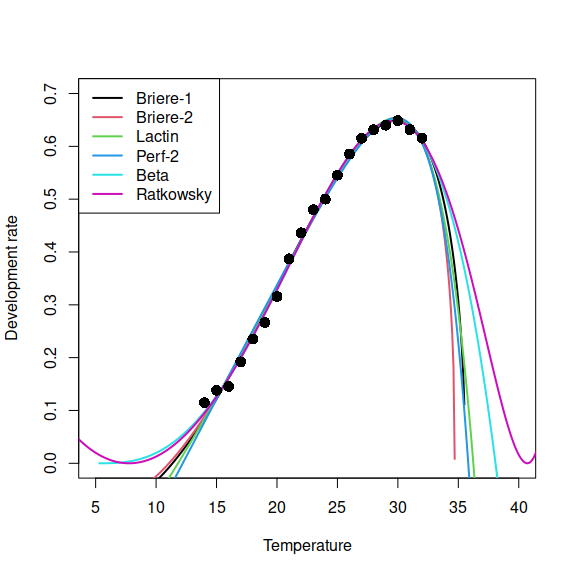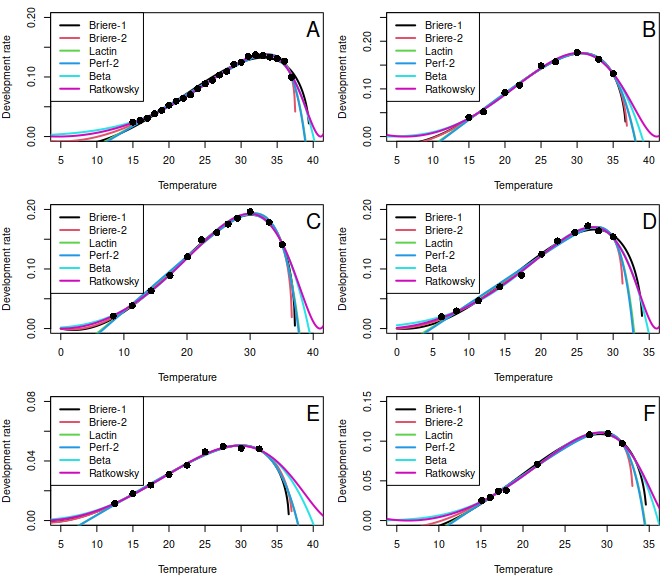# Model evaluation

#### 2023-08-24

This vignette exemplifies the use of the devRate package i) to fit a development rate models to empirical datasets with eight species using six nonlinear models, and ii) to compare models based on goodness of fit and the trade-off between the model’s goodness of fit and its structural complexity.

It reproduces some of the results of the study by Shi et al. 20161.

require("devRate")

## Nonlinear models

Table 1. Models used by Shi et al. 2016

Model name Model name in devRate
Briere-1 briere1_99
Briere-2 briere2_99
Lactin lactin2_95
Performance-2 perf2_11
Beta beta_16
Ratkowsky ratkowsky_83

## Datasets

Table 2. Datasets of temperature-dependent development rates used by Shi et al. 2016 and method used to retrieve the source dataset.

Sample species Source Method
Helicoverpa armigera Wu et al. (2009) IPEC manual
Kampimodromus aberrans Broufas et al. (2007) Table 3
Toxorhyynchites brevipalpis Trpis (1972) Table 1
Bactrocera dorsalis Messenger and Flitters (1958) Table 1
Lipaphis erysimi Liu and Meng (2000) Table 1
Myzus persicae Liu and Meng (1999) Table 1
Epilachna varivestis Shirai and Yara (2001) Table 4
Drosophila buzzatii de Jong (2010) Web Plot Digitizer

Gilpin and McClelland (1979), and Xiang et al. (2007) articles were not found and were excluded from this analysis.

wuDS <- data.frame(
temp = seq(from = 15, to = 37, by = 1),
devRate = 1/c(41.24, 37.16, 32.47, 26.22, 22.71, 19.01, 16.79, 15.63, 14.27, 12.48, 11.3,
10.56, 9.69, 9.14, 8.24, 8.02, 7.43, 7.27, 7.35, 7.49, 7.63, 7.9, 10.03))

The Broufas et al. (2007) dataset was retrieved from Table 3 where the mean development period is provided in hours and transformed in the inverse of days.

broufasDS <- data.frame(
temp = c(15.0, 17.0, 20.0, 22.0, 25.0, 27.0, 30.0, 33.0, 35.0),
devRate = 1/(c(595.4, 463.0, 260.5, 222.5, 161.8, 153.1, 136.0, 147.8, 182.1)/24))

The Trpis et al. (2007) dataset was retrieved from Table 1 where the mean egg development time is provided in hours and transformed in the inverse of days.

trpisDS <- data.frame(
temp = c(14.0, 15.0, 16.0, 17.0, 18.0, 19.0, 20.0, 21.0, 22.0, 23.0, 24.0, 25.0, 26.0,
27.0, 28.0, 29.0, 30.0, 31.0, 32.0),
devRate = 1/(c(209.0, 174.0, 165.0, 125.0, 102.0, 90.0, 76.0, 62.0, 55.0, 50.0, 48.0,
44.0, 41.0, 39.0, 38.0, 37.5, 37.0, 38.0, 39.0)/24))

The Messenger and Flitters (1958) dataset was retrieved from Table 1 where the temperature is in Fahrenheit and transformed into Celsius and the mean egg development time is provided in hours and transformed in the inverse of days.

messengerDS <- data.frame(
temp = (c(55.0, 56.0, 57.0, 58.0, 60.0, 62.5, 65.0, 67.5, 70.0, 75.0, 80.0, 85.0, 87.5,
90.0, 92.5, 95.0, 96.0, 97.0, 97.5) - 32)/1.8,
devRate = 1/(c(263.0, 232.0, 170.5, 148.0, 121.3, 95.5, 74.0, 62.5, 51.5, 38.0, 30.5,
27.0, 25.0, 24.0, 23.5, 25.0, 26.5, 29.3, 34.3)/24))

The Liu and Meng (2000) dataset was retrieved from Table 1 (constant temperatures), and for the altae form (this may be different from the dataset used by Shi et al. 2016).

liu1DS <- data.frame(
temp = c(8.3, 11.3, 14.3, 17.3, 20.1, 22.3, 24.7, 26.5, 28.0, 30.0, 33.0, 35.1),
devRate = 1/c(47.5, 26.1, 15.8, 11.2, 8.3, 6.7, 6.2, 5.7, 5.4, 5.1, 5.6, 7.1))

The Liu and Meng (1999) dataset was retrieved from Table 1 for the altae form (this may be different from the dataset used by Shi et al. 2016).

liu2DS <- data.frame(
temp = c(6.2, 8.3, 11.3, 14.3, 17.3, 20.1, 22.3, 24.7, 26.5, 28.0, 30.0),
devRate = 1/c(50.4, 33.9, 21.5, 14.3, 11.2, 8.0, 6.8, 6.2, 5.8, 6.1, 6.5))

The Shirai and Yara (2001) dataset was retrieved from Table 4 (this may be different from the dataset used by Shi et al. 2016).

shiraiDS <- data.frame(
temp = c(12.5, 15.0, 17.5, 20.0, 22.5, 25.0, 27.5, 30.0, 32.5),
devRate = 1/c(87.0, 55.5, 41.9, 32.4, 26.9, 21.7, 20.1, 20.6, 20.8))

The de Jong (2010) dataset was retrieved using Web Plot Digitizer on Figure 1B (this should be different from the dataset used by Shi et al. 2016).

deJongDS <- data.frame(
temp = c(15.1, 16.1, 17.1, 18.0, 21.7, 27.9, 30.1, 31.8),
devRate = c(0.0253, 0.0291, 0.0370, 0.0381, 0.0710, 0.1079, 0.1098, 0.0972))

## Fitting the six models to the eight empirical datasets

###  Briere-1 model

Starting values for the NLS fit were chosen on the basis of available information on the package database with the briere1_99 object.

The devRateModel function can be applied to each dataset, or a list containing all the datasets can be built to ease the process. In the first case:

wuDS_briere1_99 <- devRateModel(eq = briere1_99,
dfData = wuDS,
startValues = list(aa = 0.01, Tmin = 10, Tmax = 40))

broufasDS_briere1_99 <- devRateModel(eq = briere1_99,
dfData = broufasDS,
startValues = list(aa = 0.01, Tmin = 10, Tmax = 40))

trpisDS_briere1_99 <- devRateModel(eq = briere1_99,
dfData = trpisDS,
startValues = list(aa = 0.01, Tmin = 10, Tmax = 40))

messengerDS_briere1_99 <- devRateModel(eq = briere1_99,
dfData = messengerDS,
startValues = list(aa = 0.01, Tmin = 10, Tmax = 40))

liu1DS_briere1_99 <- devRateModel(eq = briere1_99,
dfData = liu1DS,
startValues = list(aa = 0.01, Tmin = 10, Tmax = 40))

liu2DS_briere1_99 <- devRateModel(eq = briere1_99,
dfData = liu2DS,
startValues = list(aa = 0.01, Tmin = 10, Tmax = 40))

shiraiDS_briere1_99 <- devRateModel(eq = briere1_99,
dfData = shiraiDS,
startValues = list(aa = 0.01, Tmin = 10, Tmax = 40))

deJongDS_briere1_99 <- devRateModel(eq = briere1_99,
dfData = deJongDS,
startValues = list(aa = 0.01, Tmin = 10, Tmax = 40))

The results can then be stored in a single list.

briere1NLS <- list(wuDS_briere1_99, broufasDS_briere1_99, trpisDS_briere1_99,
messengerDS_briere1_99, liu1DS_briere1_99, liu2DS_briere1_99,
shiraiDS_briere1_99, deJongDS_briere1_99)

Or alternatively:

listDS <- list(wuDS, broufasDS, trpisDS, messengerDS, liu1DS, liu2DS, shiraiDS, deJongDS)
briere1NLS <- lapply(listDS, function(myDataSet){
devRateModel(eq = briere1_99,
dfData = myDataSet,
startValues = list(aa = 0.01, Tmin = 10, Tmax = 40)
)
})

###  Briere-2 model

Likewise, the briere2_99 model can be fitted to the dataset each one at a time, or using the lapply function.

broufasDS_briere2_99 <- devRateModel(eq = briere2_99,
dfData = broufasDS,
startValues = list(aa = 0.01, Tmin = 10, Tmax = 40, bb = 2))
### and so on ...
briere2NLS <- lapply(listDS, function(myDataSet){
devRateModel(eq = briere2_99,
dfData = myDataSet,
startValues = list(aa = 0.01, Tmin = 10, Tmax = 40, bb = 2)
)
})

###  Lactin model

broufasDS_lactin2_95 <- devRateModel(eq = lactin2_95,
dfData = broufasDS,
startValues = list(aa = 0.03, Tmax = 30, deltaT = 5.0, bb = -1.5))
### and so on ...
lactin2NLS <- lapply(listDS, function(myDataSet){
devRateModel(eq = lactin2_95,
dfData = myDataSet,
startValues = list(aa = 0.03, Tmax = 30, deltaT = 5.0, bb = -1.5)
)
})

###  Performance-2 Model

perf2NLS <- lapply(listDS, function(myDataSet){
devRateModel(eq = perf2_11,
dfData = myDataSet,
startValues = list(cc = 0.02, T1 = 10, k = 0.5, T2 = 35)
)
})

###  Beta model

betaNLS <- lapply(listDS, function(myDataSet){
devRateModel(eq = beta_16,
dfData = myDataSet,
startValues = list(rm = 0.2, T1 = 5, T2 = 40, Tm = 30)
)
})

###  Ratkowsky model

ratkowskyNLS <- lapply(listDS, function(myDataSet){
devRateModel(eq = ratkowsky_83,
dfData = myDataSet,
startValues = list(cc = 0.02, T1 = 5, T2 = 40, k = 0.2)
)
})

### Organizing models’ results

Model results can be grouped into a single object to ease the data processing.

listNLS <- list(briere1NLS, briere2NLS, lactin2NLS, perf2NLS, betaNLS, ratkowskyNLS)

## Model evaluation

Shi et al. 2016 used the RSS, R2, R2 adjusted, RMSE, and AIC corrected in their study.

The RSS can be computed from the nls objects returned by the devRateModel function, and equation (7) from Shi et al. 2016.

$\begin{equation} RSS = \sum_{i=1}^n (obs_i - pred_i)^2 \end{equation}$

RSS <- t(sapply(1:6, function(myModel){
sapply(1:8, function(myDS){
return(sum((listDS[[myDS]][,2] - predict(listNLS[[myModel]][[myDS]]))^2))
})
}))
rownames(RSS) <- c("Briere-1", "Briere-2", "Lactin", "Perf-2", "Beta", "Ratkowsky")
colnames(RSS) <- c("wu", "broufas", "trpis", "messenger", "liu1", "liu2", "shirai", "deJong")

wu broufas trpis messenger liu1 liu2 shirai deJong
Briere-1 0.0004788 0.0001129 0.0032660 0.0246757 0.0001172 0.0002833 1.03e-05 4.51e-05
Briere-2 0.0001240 0.0001113 0.0031075 0.0016649 0.0000976 0.0001719 1.02e-05 2.57e-05
Lactin 0.0001841 0.0001299 0.0038827 0.0048035 0.0001950 0.0003446 1.29e-05 3.49e-05
Perf-2 0.0002115 0.0001375 0.0048966 0.0052585 0.0002361 0.0004087 1.31e-05 3.69e-05
Beta 0.0001187 0.0000953 0.0019916 0.0155084 0.0001275 0.0000839 8.60e-06 2.00e-05
Ratkowsky 0.0001054 0.0000975 0.0021200 0.0111390 0.0001203 0.0001057 8.60e-06 2.18e-05

###  R squared

The R2 can be computed using equation (8) from Shi et al. 2016.

$\begin{equation} R^2 = 1 - \frac{\sum_{i=1}^n (obs_i - pred_i)^2}{\sum_{i=1}^n (obs_i - meanObs_i)^2} \end{equation}$

R2 <- t(sapply(1:6, function(myModel){
sapply(1:8, function(myDS){
return(1 - sum((listDS[[myDS]][,2] - predict(listNLS[[myModel]][[myDS]]))^2) /
sum((listDS[[myDS]][,2] - mean(listDS[[myDS]][,2]))^2))
})
}))
rownames(R2) <- c("Briere-1", "Briere-2", "Lactin", "Perf-2", "Beta", "Ratkowsky")
colnames(R2) <- c("wu", "broufas", "trpis", "messenger", "liu1", "liu2", "shirai", "deJong")

Table 4. R2 results

wu broufas trpis messenger liu1 liu2 shirai deJong
Briere-1 0.9859933 0.9941166 0.9952558 0.9886810 0.9970427 0.9915538 0.9938871 0.9951418
Briere-2 0.9963717 0.9942003 0.9954862 0.9992363 0.9975375 0.9948735 0.9939267 0.9972306
Lactin 0.9946136 0.9932272 0.9943601 0.9977966 0.9950798 0.9897252 0.9923072 0.9962478
Perf-2 0.9938124 0.9928342 0.9928873 0.9975878 0.9940448 0.9878151 0.9922152 0.9960234
Beta 0.9965280 0.9950336 0.9971070 0.9928861 0.9967830 0.9974972 0.9948750 0.9978472
Ratkowsky 0.9969156 0.9949172 0.9969205 0.9948904 0.9969638 0.9968489 0.9948778 0.9976544

The R2 adjusted can be computed using equation (9) from Shi et al. 2016.

$\begin{equation} R^2_{adj} = 1 - \frac{n-1}{n-p}(1-R^2) \end{equation}$

R2adj <- t(sapply(1:6, function(myModel){
p <- 1 + length(coef(listNLS[[myModel]][]))
sapply(1:8, function(myDS){
n <- length(listDS[[myDS]][,2])
Rsq <- 1 - sum((listDS[[myDS]][,2] - predict(listNLS[[myModel]][[myDS]]))^2) /
sum((listDS[[myDS]][,2] - mean(listDS[[myDS]][,2]))^2)
return(1 - (n - 1)/(n - p) * (1 - Rsq))
})
}))
rownames(R2adj) <- c("Briere-1", "Briere-2", "Lactin", "Perf-2", "Beta", "Ratkowsky")
colnames(R2adj) <- c("wu", "broufas", "trpis", "messenger", "liu1", "liu2", "shirai", "deJong")

wu broufas trpis messenger liu1 liu2 shirai deJong
Briere-1 0.9837817 0.9905866 0.9943070 0.9864172 0.9959338 0.9879340 0.9902193 0.9914982
Briere-2 0.9955654 0.9884006 0.9941965 0.9990181 0.9961304 0.9914558 0.9878535 0.9935380
Lactin 0.9934167 0.9864544 0.9927487 0.9971670 0.9922683 0.9828754 0.9846144 0.9912450
Perf-2 0.9924374 0.9856684 0.9908551 0.9968987 0.9906418 0.9796918 0.9844304 0.9907213
Beta 0.9957564 0.9900672 0.9962804 0.9908536 0.9949447 0.9958287 0.9897499 0.9949768
Ratkowsky 0.9962301 0.9898344 0.9960407 0.9934305 0.9952288 0.9947482 0.9897556 0.9945270

###  RMSE

The RMSE can be computed using equation (10) from Shi et al. 2016.

$\begin{equation} RMSE = \sqrt{RSS/(n-p+1)} \end{equation}$

RMSE <- t(sapply(1:6, function(myModel){
p <- 1 + length(coef(listNLS[[myModel]][]))
sapply(1:8, function(myDS){
n <- length(listDS[[myDS]][,2])
return(sqrt(RSS / (n - p + 1)))
})
}))
rownames(RMSE) <- c("Briere-1", "Briere-2", "Lactin", "Perf-2", "Beta", "Ratkowsky")
colnames(RMSE) <- c("wu", "broufas", "trpis", "messenger", "liu1", "liu2", "shirai", "deJong")

Table 6. RMSE results

wu broufas trpis messenger liu1 liu2 shirai deJong
Briere-1 0.0048931 0.0043370 0.0142873 0.0392713 0.0036089 0.0059508 0.0013088 0.0030044
Briere-2 0.0025551 0.0047171 0.0143932 0.0105354 0.0034930 0.0049562 0.0014291 0.0025362
Lactin 0.0031131 0.0050975 0.0160886 0.0178950 0.0049374 0.0070166 0.0016084 0.0029520
Perf-2 0.0033367 0.0052433 0.0180677 0.0187235 0.0054320 0.0076410 0.0016180 0.0030390
Beta 0.0024994 0.0043651 0.0115229 0.0321542 0.0039924 0.0034630 0.0013128 0.0022361
Ratkowsky 0.0023558 0.0044159 0.0118884 0.0272507 0.0038786 0.0038857 0.0013124 0.0023340

###  AIC corrected

The AICc can be computed using equations (11) and (12) from Shi et al. 2016.

$\begin{equation} AIC_c = -2L+2pn/(n-p-1) \end{equation}$

$\begin{equation} L = -\frac{n}{2}ln\frac{RSS}{n} \end{equation}$

AICc <- t(sapply(1:6, function(myModel){
p <- 1 + length(coef(listNLS[[myModel]][]))
sapply(1:8, function(myDS){
n <- length(listDS[[myDS]][,2])
L <- -n/2 * log(RSS / n)
return(-2 * L + 2 * p * n / (n - p - 1))
})
}))
# number of parameters + 1
p <- sapply(1:6, function(myModel){
p <- 1 + length(coef(listNLS[[myModel]][]))
return(p)
})
# sample size
n <- sapply(1:8, function(myDS){
n <- length(listDS[[myDS]][,2])
return(n)
})
rownames(AICc) <- paste0(c("Briere-1", "Briere-2", "Lactin",
"Perf-2", "Beta", "Ratkowsky"), " (", p, ")")
colnames(AICc) <- paste0(c("wu", "broufas", "trpis", "messenger",
"liu1", "liu2", "shirai", "deJong"), " (", n, ")")

Table 7. AICc results with the number of parameters + 1 in parenthesis next to the model names and the sample size in parenthesis next to the sample names.

wu (23) broufas (9) trpis (19) messenger (19) liu1 (12) liu2 (11) shirai (9) deJong (8)
Briere-1 (4) -237.7094 -83.57931 -153.8467 -115.4240 -124.7222 -101.56951 -105.14419 -75.34930
Briere-2 (5) -265.4701 -71.70819 -151.0340 -162.8907 -120.6337 -99.72838 -93.20277 -61.17890
Lactin (5) -256.3825 -70.31223 -146.8024 -142.7588 -112.3275 -92.08044 -91.07526 -58.74932
Perf-2 (5) -253.1931 -69.80455 -142.3940 -141.0391 -110.0364 -90.20487 -90.96829 -58.28460
Beta (5) -266.4824 -73.10415 -159.4861 -120.4901 -117.4263 -107.61567 -94.73057 -63.19385
Ratkowsky (5) -269.2051 -72.89570 -158.2993 -126.7777 -118.1202 -105.08191 -94.73557 -62.50768

###  AIC

The AIC can also be computed with the AIC function in the stats package.

AIC <- t(sapply(1:6, function(myModel){
sapply(1:8, function(myDS){
return(AIC(listNLS[[myModel]][[myDS]]))
})
}))
rownames(AIC) <- c("Briere-1", "Briere-2", "Lactin", "Perf-2", "Beta", "Ratkowsky")
colnames(AIC) <- c("wu", "broufas", "trpis", "messenger", "liu1", "liu2", "shirai", "deJong")

Table 8. AIC results

wu broufas trpis messenger liu1 liu2 shirai deJong
Briere-1 -174.6604 -68.03842 -102.78414 -64.36146 -96.38192 -77.01953 -89.60330 -65.97961
Briere-2 -203.7283 -66.16729 -101.72973 -113.58638 -96.57913 -80.51174 -87.66187 -68.47588
Lactin -194.6407 -64.77133 -97.49810 -93.45456 -88.27294 -72.86379 -85.53437 -66.04631
Perf-2 -191.4513 -64.26366 -93.08971 -91.73482 -85.98187 -70.98822 -85.42739 -65.58158
Beta -204.7406 -67.56325 -110.18178 -71.18581 -93.37173 -88.39903 -89.18968 -70.49084
Ratkowsky -207.4633 -67.35481 -108.99506 -77.47345 -94.06567 -85.86526 -89.19468 -69.80466

## Visualization

The fitted models can be plotted using the devRatePlot function from the devRate package, or alternatively they can be plotted using the predict function from the stats package. In the following code, listDS[] refers to the fourth dataset from Messenger and Flitters (1958) and listNLS[[i]][] refers to the corresponding model fit.

plot(x = listDS[][,1],
y = listDS[][,2],
ylim = c(0, 1), ylab = "Development rate",
xlim = c(5, 40), xlab = "Temperature", type = "n")
for(i in 1:6){
points(x = seq(from = 0, to = 50, by = 0.1),
y = predict(listNLS[[i]][], newdata = list(T = seq(from = 0, to = 50, by = 0.1))),
type = 'l', lwd = 2, col = i)
}
points(x = listDS[][,1], y = listDS[][,2], pch = 16, cex = 1.5)
legend("topleft", col = 1:6, lwd = 2, legend = c("Briere-1", "Briere-2", "Lactin", "Perf-2", "Beta", "Ratkowsky"))Figure 1. The six models fitted to the Messenger and Flitters (1958) dataset.

plot(x = listDS[][,1],
y = listDS[][,2],
ylim = c(0, 0.7), ylab = "Development rate",
xlim = c(5, 40), xlab = "Temperature", type = "n")
for(i in 1:6){
points(x = seq(from = 0, to = 50, by = 0.1),
y = predict(listNLS[[i]][], newdata = list(T = seq(from = 0, to = 50, by = 0.1))),
type = 'l', lwd = 2, col = i)
}
points(x = listDS[][,1], y = listDS[][,2], pch = 16, cex = 1.5)
legend("topleft", col = 1:6, lwd = 2, legend = c("Briere-1", "Briere-2", "Lactin", "Perf-2", "Beta", "Ratkowsky"))Figure 2. The six models fitted to the Trpis (1972) dataset.

getPlot <- function(datasetNumber, ...){
plot(x = listDS[[datasetNumber]][,1],
y = listDS[[datasetNumber]][,2],
ylab = "Development rate",
xlab = "Temperature", type = "n", ...)
for(i in 1:6){
points(x = seq(from = 0, to = 50, by = 0.1),
y = predict(listNLS[[i]][[datasetNumber]], newdata = list(T = seq(from = 0, to = 50, by = 0.1))),
type = 'l', lwd = 2, col = i)
}
points(x = listDS[[datasetNumber]][,1], y = listDS[[datasetNumber]][,2], pch = 16, cex = 1.5)
legend("topleft", col = 1:6, lwd = 2,
legend = c("Briere-1", "Briere-2", "Lactin", "Perf-2", "Beta", "Ratkowsky"))
}
par(mfrow = c(3, 2), mar = c(4, 4, 1, 1))
getPlot(datasetNumber = 1, ylim = c(0, 0.20), xlim = c(5, 40))
text(x = 40, y = 0.20, labels = "A", cex = 2, pos = 1)
getPlot(datasetNumber = 2, ylim = c(0, 0.25), xlim = c(5, 40))
text(x = 40, y = 0.25, labels = "B", cex = 2, pos = 1)
getPlot(datasetNumber = 5, ylim = c(0, 0.20), xlim = c(0, 40))
text(x = 40, y = 0.20, labels = "C", cex = 2, pos = 1)
getPlot(datasetNumber = 6, ylim = c(0, 0.20), xlim = c(0, 35))
text(x = 35, y = 0.20, labels = "D", cex = 2, pos = 1)
getPlot(datasetNumber = 7, ylim = c(0, 0.08), xlim = c(5, 40))
text(x = 40, y = 0.08, labels = "E", cex = 2, pos = 1)
getPlot(datasetNumber = 8, ylim = c(0, 0.15), xlim = c(5, 35))
text(x = 35, y = 0.15, labels = "F", cex = 2, pos = 1)Figure 3. The six models fitted to the other datasets: A: Wu et al. (2009), B: Broufas et al. (2007), C: Liu and Meng (2000), D: Liu and Meng (1999), E: Shirai and Yara (2001), and F: de Jong (2010).

## Conclusion

The first four datasets gave the same results as in Shi et al. 2016, and the last four different results, probably because the datasets retrieved from the source may differ (e.g., altae or apterae aphid data in Liu et al. 1999; 2000). This vignette exemplifies the use of the devRate package to compare models. For interpretations, see the extensive literature on model evaluation with RSS, R2, R2 adjusted, RMSE, AIC, AIC corrected available online, and the study by Shi et al. 2016.

1. Shi, P. J., Reddy, G. V., Chen, L., & Ge, F. (2015). Comparison of thermal performance equations in describing temperature-dependent developmental rates of insects:(I) empirical models. Annals of the Entomological Society of America, 109(2), 211-215.↩︎